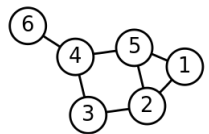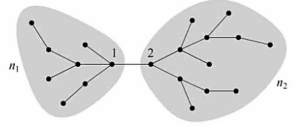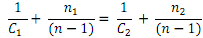+1-415-670-9189
info@expertsmind.com

# Get Solution

Compute degree centrality of an undirected graph
Course:- Software Engineering
Reference No.:- EM13318245

 TweetExpertsmind Rated 4.9 / 5 based on 47215 reviews.
Review Site
Assignment Help >> Software Engineering

1. Compute the degree centrality, betweeness centrality, closeness centrality of nodes in the following graph.2. A k-regular undirected network is a network in which every vertex has degree ??. Show that the vector ?? = (1, 1, 1, . . . , 1) is an eigenvector of the adjacency matrix with eigenvalue ??.

3. Consider an undirected tree of n vertices. A particular edge in the tree joins vertices 1 and 2 and divides the tree into two disjoint regions of n1 and n2 vertices as sketched here:Show that the closeness centralities ??1 and ??2 of the two vertices are related by4. Calculate the betweenness centrality of the ??th vertex from the end of a "line graph" of n vertices. Here a line graph on n vertices 1, 2, 3,..., n has exactly n - 1 edges that connect vertices ?? and ?? + 1, for ?? = 1,..., n - 1.5. Write a program in your preferred programming language to compute degree centrality of an undirected graph. The program will read the graph from a file called "graph.txt" and output the degree centrality of nodes to a file called "degree.txt".

The file "graph.txt" includes multiples lines in which the first line contains two integers n and m that correspond to the number of nodes and edges in the graph. Each of the following m lines contain two integers u and v, separated by one space, to denote an edge from u to v. Nodes are numbered from 1 to n.

The output file "degree.txt" contains exactly n lines in which the ????h line is the (unnormalized) degree centrality of node ??.

Your submission must include
- The source file(s)
- The sample input/output
- A README file that describes the compile and running instruction

6. Write a program in your preferred programming language to compute (normalized) closeness centrality of an undirected graph. The program will read the graph from a file called "graph.txt" and output the degree centrality of nodes to a file called "closeness.txt".

The file "graph.txt" includes multiples lines in which the first line contains two integers n and m that correspond to the number of nodes and edges in the graph. Each of the following m lines contain two integers u and v, separated by one space, to denote an edge from u to v. Nodes are numbered from 1 to n.

The output file "closeness.txt" contains exactly n lines in which the ????h line is the (normalized) closeness centrality of node ??.

Your submission must include

- The source file(s)
- The sample input/output
- A README file that describes the compile and running instruction

Minimize

Ask Question & Get Answers from Experts
Browse some more (Software Engineering) Materials
 Make a pseudocode algorithm you would write for a simple task like making a turkey sandwich, as well as 3-simple control structures that could be used to make this algorithm. Provide the UML diagrams for the given problem with clear explanations on the design decisions. Derive detailed Use Case diagram, Class diagram & a sequence diagram and prov Define a class called Counter. An object of this class is used to count things so it records a count that is a non-negative whole number. Include methods to set the counter Taylor's Professional Services is a technical and engineering staffing service.  When a TPS client company determines that it will need a temporary professional or scientifi Differentiate between alpa and beta testing, Consider a piece of an embedded software that is a part of a T.V. Which of the types of system testing would you choose to apply a In a language without exception-handling facilities, we could send an error-handling process as a parameter to each process that can detect errors that must be handled. Design and list 10 test cases and explain the purpose of each of them in 1-2 sentences. (e.g., the purpose of [3, 4, 5] is to test that the program correctly identifies a ri Developing the prime number project and build a debug version of the executable randomPrime; your task is to develop alternative programs with different functionality and to